StatLect

# Variance formula

A variance formula is an equation used to compute or define the variance.

There are several formulae that can be used, depending on the situation.## General formula

We start with a general formula, used to define the variance of a random variable:where:

•denotes the variance;

•denotes the expected value operator.

This is a definition and it is useful because of its generality. However, if you need to calculate the variance in practice, you need to use the equations below.

## Formula for discrete variables

When the random variable is discrete the above formula becomes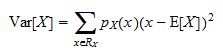where:

•is the set of all possible realizations of(called the support of);

•is the probability mass function of;

• the notationindicates that we need to perform a sum over all the values thatcan take.

In other words, we need to compute a weighted average of the squared deviations offrom its mean.

### Example

Suppose thatcan take only two values,and, each with probability.

Then, the support ofisand its probability mass function is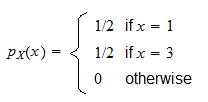We first need to calculate the expected value: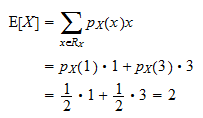Then, we can compute the variance: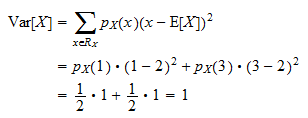## Formula for continuous variables

Whenis continuous, the variance formula is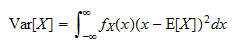where:

•is the probability density function of;

• the notationindicates that we need to take an integral betweenand.

To understand the next example you need to be familiar with integration rules.

### Example

Suppose thathas a uniform distribution, with probability densityWe compute the expected value: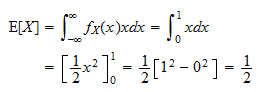The variance is: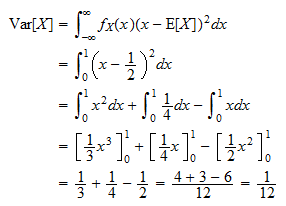## Variance formula based on moments

Instead of computing variance using the formulae above, it is often easier to use the following equivalent equation based on moments:### Example

Let us continue with the example of the uniform distribution.

Instead of using the variance formula for continuous variables, we compute the first moment(already done!) and the second moment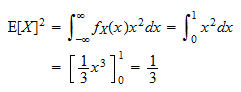Then, we plug the two moments in the equation: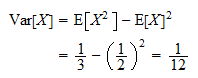### Use with moment generating function

When we know the moment generating function of, we can use it to compute the two momentsandand then plug their values in the formula above.

## Formulae for the sample variance

Until now, we have discussed how to calculate the variance of a random variable.

However, there is another concept, that of sample variance, that applies when we need to assess the dispersion of some observations around their sample mean.

If you are interested in the formulae for sample variance, you can check the following pages:

## More details, proofs and exercises

More details about these formulae - including proofs and solved exercises - can be found in the lecture entitled Variance.

Previous entry: Unbiased estimator